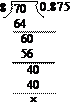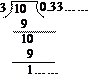# Mathematics

### Chapter : Real Number

#### Number System - Irrational number

Irrational number :
(i) A number is irrational if and only if its decimal representation is non-terminating and non-repeating. e.g., , &sqrt2, &sqrt5, π etc.
(ii) Rational number and irrational number taken together form the set of real numbers.
(iii) If a and b are two real numbers, then either
(i) a > b or (ii) a = b or (iii) a < b
(iv) Negative of an irrational number is an irrational number.
(v) The sum of a rational number with an irrational number is always irrational.
(vi) The product of a non-zero rational number with an irrational number is always an irrational number.
(vii) The sum of two irrational numbers is not always an irrational number.
(viii) The product of two irrational numbers is not always an irrational number.
In division for all rationals of the form (q ≠ 0), p & q are integers, two things can happen either the remainder becomes zero or never becomes zero. Some of the solved examples are
Type (1) : 7/8 = 0.875This decimal expansion 0.875 is called terminating. Therefore this decimal expansion 0.875 is called terminating.
If remainder is zero then decimal expansion ends (terminates) after finite number of steps. These decimal expansion of such numbers terminating.Type (2) : 1/3 = 0.333……… =Take another example 1/7 = 0.142857142857… =In both examples remainder is never becomes zero so the decimal expansion is never ends after some or infinite steps of division. These type of decimal expansions are called non terminating . In above examples, after Ist step & 6 steps of division (respectively) we get remainder equal to dividend so decimal expansion is repeating (recurring). So these are called a non terminating recurring decimal expansions . Both the above types (1 & 2) are rational numbers.
Types (3) : Some of the decimal expansion 0.327172398……is not ends any where, also there is no arrangement of digits (not repeating) so these are called non terminating not recurring . These numbers are called irrational numbers.
0.1279312793 rational terminating 0.1279312793…. rational non terminating or& recurring 0.32777 rational terminating or rational non terminating 0.32777……. & recurring 0.5361279 rational terminating 0.3712854043…. irrational non terminating non recurring 0.10100100010000 rational terminating 0.10100100010000…. irrational non terminating non recurring.